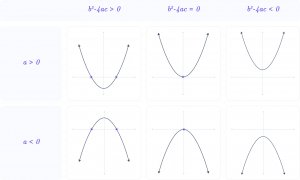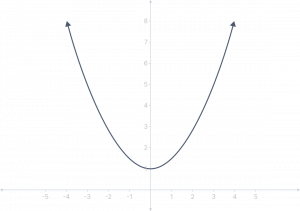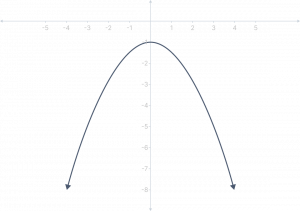A quadratic Calculator is used to find the roots of the given quadratic equation. Our online quadratic formula calculator tool speeds up the computation and shows the roots in a snap.

Please provide values for $$ax^2 + bx + c = 0$$

A quadratic equation is a polynomial equation whose highest degree is 2. When graphed, quadratic equations have the shape of parabolas.

$$ax^2 + bx + c = 0$$ is the quadratic equation’s conventional form.

Where A, B, and C are numerical values, A shouldn’t be equal to 0, and X is a variable. An example of quadratic equation is $$3x^2 + 24x + 2 = 0$$

The root of the equation is the value of the variable x.

Finding the values at which a quadratic is zero is commonly important in algebraic situations. In other words, it’s essential to identify the zeros, roots, or solutions to a quadratic equation.

The quadratic formula is one of the top five mathematical formulae that aid in solving quadratic equations. Any quadratic problem can be solved using the quadratic formula. The equation is first changed to $$ax^2 + bx + c = 0$$, where a, b, and c are coefficients. Next, we enter the following coefficients into the formula:

$$x = \dfrac{-b \pm \sqrt{b^2 – 4ac}}{2a}$$

Here, $${b^2 – 4ac}$$ is discriminant, which shows the possible solutions of the equations. Although it could initially seem a little frightening, you’ll quickly adjust!## What is the Quadratic Formula used for?

The Quadratic Formula is used to find the roots of a quadratic equation. It was first discovered in antiquity, and since then, different derivations, arguments, and intuitions have been developed to explain it. Some employ geometrical techniques such as analyzing extrema. There is a tonne more, too.

## How to Use the Quadratic Formula Calculator?

Follow these steps to solve a second-order polynomial equation using our online quadratic formula calculator.

1. Put the equation’s coefficients into the appropriate input area.
2. Then, click the “Solve” button.
3. The output field will then show the quadratic equation’s roots.

## Tips & Tricks

• Factorization is typically used to solve quadratic problems. However, the quadratic formula is utilized when factorization is not an option.
• The zeroes of the equation are another name for the quadratic equation’s roots.
• In quadratic equations with negative discriminant values, complex numbers are used to represent the roots.
• It can be done using their sum and product to find higher algebraic expressions involving the roots of a quadratic equation.

## Concave Up Parabola (Cup-up Parabola)## Concave Down Parabola (Cup-down Parabola)